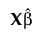Publication date: 04/12/2021

##Profilers

The plots in the Marginal Model Profiler are based on marginal predicted values. These are the predicted values obtained if you select Save Columns > Prediction Formula.

Denote the linear mixed model by E[Y|γ] = Xβ + Zγ. Here β is the vector of fixed effect coefficients and γ is the vector of random effect coefficients. The marginal predictions are the predictions from the fixed effects part of the predictive model, given by.

Note: Marginal model profiler plots show predictions for distinct settings of the fixed effects only. The Profiler shows only cells corresponding to fixed effects. In other profilers, where random effects can be displayed, only the settings of the fixed effects determine the predicted values.

Four types of profilers are provided:

Profiler

Contour Profiler

Mixture Profiler

Surface Profiler

Options that are appropriate for the model that you are fitting are enabled. See Figure 8.21 for an example of a profiler. See Figure 8.42 for an example of a Surface Profiler. For more information about the surface profiler, see Surface Plot in Profilers.

Want more information? Have questions? Get answers in the JMP User Community (community.jmp.com).